# Fibonacci in Scheme

I used Binet's Formula to calculate fibonacci numbers:

(define (fib n)
(/ (- (expt (/ (+ 1 (sqrt 5)) 2) n) (expt (/ (- 1 (sqrt 5)) 2) n)) (sqrt 5)))

(fib 5)


So,

(define (fib n)
(/ (- (expt (/ (+ 1 (sqrt 5)) 2) n) (expt (/ (- 1 (sqrt 5)) 2) n)) (sqrt 5)))

(define-macro for
(lambda (iterator start end step . body)
(let loop ((,iterator ,start))
(if (<= ,iterator ,end)
(loop (+ ,iterator ,step) (begin ,@body))))))

(for x -1 1 (/ 1 10)
(print "fib " x " = " (fib x)))


results in

fib -1 = 0.9999999999999998
fib -0.9 = +nan.0
fib -0.8 = +nan.0
fib -0.7000000000000001 = +nan.0
fib -0.6000000000000001 = +nan.0
fib -0.5000000000000001 = +nan.0
fib -0.40000000000000013 = +nan.0
fib -0.30000000000000016 = +nan.0
fib -0.20000000000000015 = +nan.0
fib -0.10000000000000014 = +nan.0
fib -1.3877787807814457e-16 = +nan.0
fib 0.09999999999999987 = +nan.0
fib 0.19999999999999987 = +nan.0
fib 0.2999999999999999 = +nan.0
fib 0.3999999999999999 = +nan.0
fib 0.4999999999999999 = +nan.0
fib 0.5999999999999999 = +nan.0
fib 0.6999999999999998 = +nan.0
fib 0.7999999999999998 = +nan.0
fib 0.8999999999999998 = +nan.0
fib 0.9999999999999998 = +nan.0


(in BiwaScheme, so all those 0.n99999999999999o-type things)
However, I don't really understand the +nan.0s.

Probably it means you did 0/0, or one of those EXPT calls (see why case sensitivity is such a bad idea?) is taking a negative number to a fractional power.

The only things I'm dividing by are 2 and (sqrt 5), so that's not it.

How would that work?

Yeah.

Well, I could be wrong, does that Scheme include complex numbers? Because you know in the real numbers you can't take sqrt(-1), i.e., you can't take (-1)^(1/2).

IDK. I'll have to see.

Edit: Nope:

(print (sqrt -1))

+nan.0


I still don't understand how nan can be signed, or how you can have nan.x with x as an integer. (text manipulation there, in math you can't have x.y with y an integer.)

But apparently negative numbers to fractional powers aren't forbidden: (sigh of relief)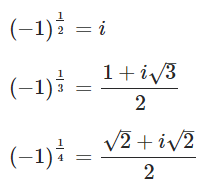# First, why did I put fib in the factorial block?

Ok. In Snap!, Bignums on, with Binet's Formula: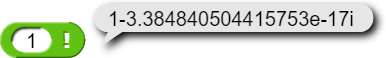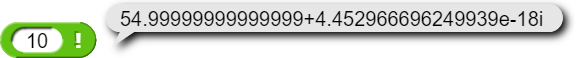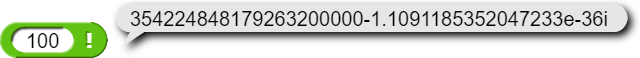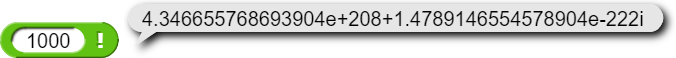I don't think those are right, and I think I've found the culprit: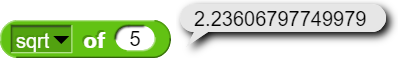Uhh...: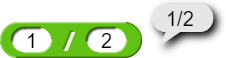as expected, but: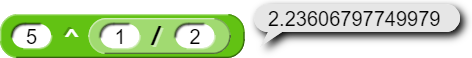Oh look:

But:

And now:
(fib 1):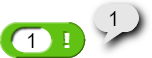(fib 10): (has a /)

(fib 100): (has a /`) (looks like a 1px line to me here)

(fib 1000):
-ugh image corrupted on creation-
(I don't think I should do (fib 10000))

>one million digits of (sqrt 5)

The issue with

is that the square root of 5 is irrational, which means that it can never be represented exactly by a fraction.
The only way you can represent the square root of 5 exactly is by writing $$\sqrt{5}$$.

All Fibonacci numbers are integers, since you calculate them by adding integers together. Using Binet's formula, all of the $$\sqrt{5}$$ just cancel out once you do the math. For example, plugging $$\frac{(\frac{1+\sqrt{5}}{2})^{100}-(\frac{1-\sqrt{5}}{2})^{100}}{\sqrt{5}}$$ into my calculator gives an exact integer, 354224848179261915075.

Okay, so, about NaN.0, I'm sure that's just an artifact of their lazy number printer, which uses the decimal point in all floating point values instead of #i. As for the sign, if you think about 0/0 as the limit of 1/1, 0.1/0.1, 0.01/0.01, etc., you get a positive NaN, but if you think of it as -1/1, -0.1/0.1, etc., you get a negative NaN.

Almost all numbers are irrational. (If you throw an infinitely fine dart at the number line, the probability of hitting a rational number is zero. (Think about that the next time someone tells you you're not going to win the \$600M Powerball tonight. At least the probability is larger than zero! (Supposing you bought a ticket.))) That means almost all numbers can't be represented exactly in a computer.

That's true, but we still shouldn't report an incorrect answer. I think I remember that it's acceptable to report the still-inexact value, but really we should give an error message. But we can't, because we no longer remember that the IEEE floating point input to EXACT came from an irrational expression. All floats are exact rationals, even if not very interesting ones.

Exactly!

Yeah. There are infinitely many irrational numbers between any two rational numbers, no matter the distance between them.

Yes. What I'd want is for ([sqrt v] of (5)) to give something like "$$\sqrt5$$".

But that's true of the rational numbers. (The name for that property is "dense," as in "the rational numbers are dense.") You can prove it by taking the average of the two given numbers, which is provably rational and provably between the two givens. Denseness isn't enough for a set to be larger than the integers. There are the same number of rational numbers as of integers, but there are (many) more irrationals than rationals, to the point where picking a point at random on the number line has a probability of zero of hitting a rational, not just "very near zero" or the like.

This is set theory, one of the coolest things in math.

Yeah, that's a symbolic algebra program (like Mathematica, Macsyma, Matlab, etc.); you can find an implementation in SICP 2.5.3.

I'm not actually sure where I'd find that.

Isn't this also true for the dart hitting a computable number? (like the square root of 5)

But do non-computable numbers "exist"? And even if they do, why should we are about them?

Why do we care about the Halting Theorem? It's interesting and useful to know the boundaries of what we can achieve. (And we care about NP problems because they delimit the boundaries of what we can achieve in practice.) I think it was Bentley who said it's worth learning asymptotic analasys of algorithms so that when your boss asks you to solve some NP-complete problem, you don't have to waste time trying.

Not to mention, it's fun!

I was echoing a view of some computer scientists that I'm sympathetic with. The Halting Theorem says something interesting about computation. What are these numbers that aren't computable? Can they be specified? Can you name one?

And sometimes when asked to solve some NP-complete problem, one can find heuristics that work for real-world problems (or are good enough). Not necessarily a waste of time.

Thanks! (lots of Scheme there, I'll have to test it out eventually)

Only nth ones are, where n is itself an integer. The rest are complex numbers.

This is reminiscent of the "interesting number" thing, but different because you can't ask for the smallest positive uncomputable number. But yes, you can describe several uncomputable numbers if unlike me you remember what you've read on this topic. :~(

We'll make you a Knight of the Lambda Calculus yet! :~)

Ok.

It seems that Chaitin's constant - Wikipedia is an example. "... halting probability is a real number that, informally speaking, represents the probability that a randomly constructed program will halt."